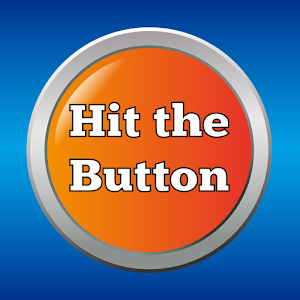# Times Tables

Martial Arts Multiplication

Children will be able to earn Martial Arts Multiplication awards once they have completed a series of challenges demonstrating their skills. These awards will be graded according to Martial Art style colour bands (linked to National Curriculum expectations).Children will be tested in school on each of the times tables (see order above). They will be given 1 minute to complete 13 questions. There will be 3 separate tests: forwards, backwards and random. On occasion, teachers may use additional activities to test understanding. Here is an example of how a typical test will look, using the 4 times tables.

Note: These may also be presented in reverse i.e. 3 x 4 = OR 4 x 3 =.

 Forwards (1 min) Backwards (1 min) Random (1 min) 0 x 4 = 1 x 4 = 2 x 4 = 3 x 4 = 4 x 4 = 5 x 4 = 6 x 4 = 7 x 4 = 8 x 4 = 9 x 4 = 10 x 4 = 11 x 4 = 12 x 4 = 12 x 4 = 11 x 4 = 10 x 4 = 9 x 4 = 8 x 4 = 7 x 4 = 6 x 4 = 5 x 4 = 4 x 4 = 3 x 4 = 2 x 4 = 1 x 4 = 0 x 4 = 5 x 4 = 2 x 4 = 3 x 4 = 11 x 4 = 4 x 4 = 6 x 4 = 9 x 4 = 1 x 4 = 10 x 4 = 0 x 4 = 7 x 4 = 12 x 4 = 8 x 4 =

The children will be given blank grids that they will have time to prepare (see below). These can then be used for whichever times tables they are currently being tested on.

You may download a copy to use at home – download here.

↑ Times Tables Resources

Maths is fun – useful lesson on times tables

Year 4 Test – example of Year 4 National Multiplication Tables Check

Daily 10 (webapp) – times tables and other quick fire questions

Coconut Multiples (webapp) – multiples linked to specific times tables

Hit the Button (webapp – free) / iOS App / Android App (paid) – times tables quiz10 Minutes a Day iOS App / Android App (free) – times tables quiz